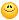About Store Forum Documentation ContactWeighted average with colours/floats problem Author Message
ChrisMember

 Post: #1 Weighted average with colours/floats problem Hi, I'm trying to compute the weighted average of some data. Originally I had a set of greyscale colours, and did: Vec avg = Vec(0); Flt count = 0; FREP(n) { Vec pos = ...; Color weight = ...; // greyscale between 0 (black) and 255 (white); avg += pos * weight.asVec(); count += weight.asVec().x; } avg /= count; This worked, however I want to use Flts now instead of colours (i'm done visualising). But for some reason I get -1.#INF000 etc for count and -1.#IND000 for avg. e.g: FREP(n) { Vec pos = ...; Flt weight = ...; avg += pos * weight; // this works if I use avg += pos * Color(Vec(weight)).asVec(); and.. count += weight; // count += Color(Vec(weight)).asVec().x; } avg /= count; I tried checking for division by zero. What is it in converting the weight to a colour byte which makes it work? I just want to understand why its not working with floats - why count and avg are -1.#INF000 when they get to the division. EDIT: Solved, I needed to check if the weight was greater than zero before multiplying it. So strange that I didn't need to do this when it was a zero colour.asVec(); Must've been guarded in EE already then ~ haven't been stuck on such a basic error as this for a looong time(This post was last modified: 11-25-2010 07:22 PM by Chris.)
11-25-2010 05:07 PM
 « Next Oldest | Next Newest »